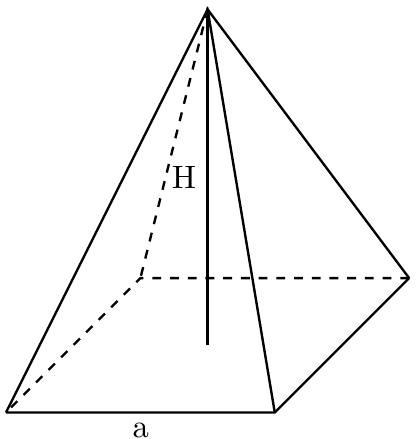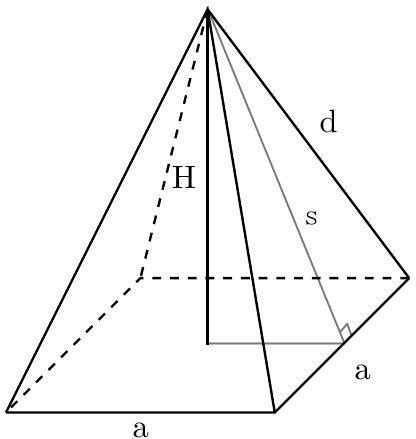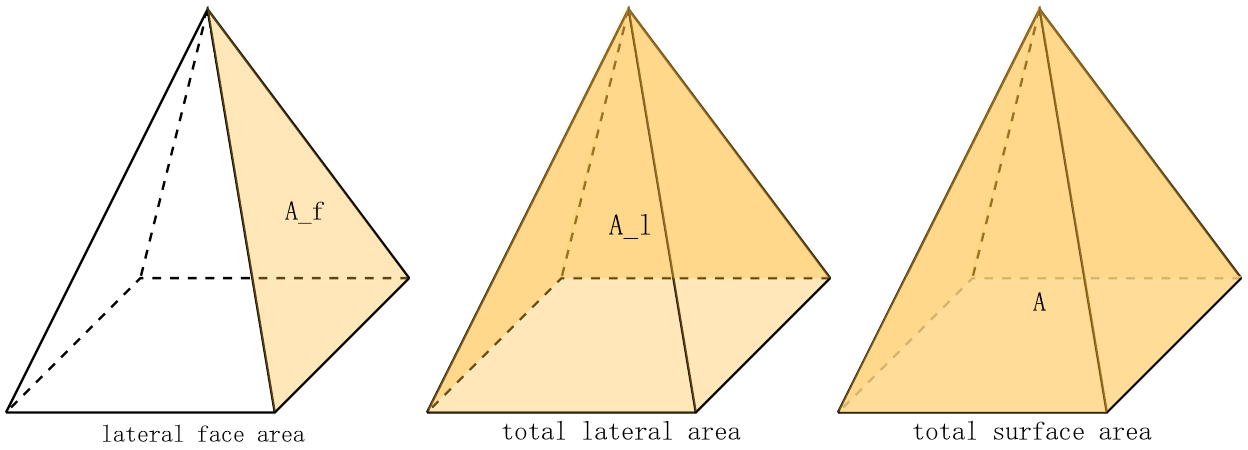# Square Pyramid Volume Calculator

Created by Kenneth Alambra
Reviewed by Madhu Raman
Last updated: Jul 27, 2022

This square pyramid volume calculator will help you learn:

• how to find the volume of a right square pyramid,
• the different square pyramid volume formulas, and
• the measurements you can and cannot use to find a square pyramid's volume.

This volume of a pyramid with a square base calculator is easy to use and comes with explanations and instructions to help you with your square pyramid volume calculations. Keep on reading to start learning 🙂!

## How to use this square pyramid volume calculator

To find the volume of a pyramid with a square base, this calculator will be your best friend. All you need to do is enter any two measurements of your square pyramid into our calculator. Once you've done that, our calculator will then provide you with the volume of your square pyramid 🙂.

In the next section of this text, we'll discuss the different square pyramid formulas we used to make this volume of a pyramid with a square base calculator work.

## How to find the volume of a right square pyramid

The formula we use to find the volume of a square pyramid only uses two variables that we can easily measure on a pyramid. Those measurements are the base edge (a) and the height (H) of the pyramid, shown in the image below:With those measurements, we can then calculate the volume of the square pyramid (V) with the help of this formula:

V = a² * H / 3

💡 It's a good thing to note that the a² part of the square pyramid volume formula actually calls for its square base area.

We can quickly determine a square pyramid's volume using the aforementioned formula. However, sometimes, we cannot measure a or H, say something obstructs us from doing so. But instead, we can measure or have at least one of the values for the slant height (s) and lateral edge (d), shown in the image below:In those cases, we can utilize the Pythagorean Theorem to express a in terms of s or d and H in terms of a and s. Then we can derive and use one of these square pyramid volume formulas, depending on the measurements we know:

If we don't know H:

• V = a² * √(s² - (a / 2)²) / 3

• V = a² * √(d² - a² / 2) / 3

If we don't know a:

• V = 4 * (s² - H²) * H / 3

• V = 4 * (d² - s²) * H / 3

• V = 2 * (d² - H²) * H / 3

If you want to learn more about finding a square pyramid's height, you can check out our height of a square pyramid calculator.

## Other ways to find the square pyramid volume

In some other scenarios, we may lack the measurements mentioned above to quickly find a square pyramid's volume. Good thing we can derive those measurements using the different surface areas of our pyramid, as shown in this image:We can find the slant height using the lateral face area if we know our pyramid's base edge. The same goes with the total lateral area, where it is simply the lateral face area multiplied by four. You can learn more about these surface areas and the formulas that you can use to derive a, s, or H in our right square pyramid calculator.

Craving for more pyramid-related calculations and knowledge? Here are some of our other cool calculators you can check out 🙂:

## FAQ

### How do you calculate the volume of a square pyramid?

Let's say we have a small pyramid with a 6-inch × 6-inch square base and a height of 10 inches. To calculate its volume:

1. First, find the area of its base, 6 in × 6 in = 36 in².
2. Then, multiply this area by the pyramid's height, 36 in² × 10 in = 360 in³.
3. Finally, divide this product by 3 to get the volume, 360 in³ / 3 = 120 in³.

### What is the volume of a regular square pyramid formula?

You can find the volume of a regular square pyramid using the formula volume = base area × height / 3. Take note that the base area equals the square of the pyramid's base edge length. On the other hand, the height is the perpendicular distance between the pyramid's base and the pyramid's vertex.

### What is the volume of the Great Pyramid of Giza?

The Great Pyramid of Giza's volume was around 2.6 million m³ (92 million ft³). Originally, including its smooth limestone casing, the Great Pyramid had a height of 146.7 m (481.4 ft) and a base edge length of 230.6 m (756.4 ft). Using these values and the square pyramid volume, we obtained its volume, V = 230.6² × 146.7 / 3 = 2600324.004 m³ ≈ 2.6 million m³.

Kenneth Alambra
This calculator can find the volume of any right square pyramid. Enter any two of your pyramid's dimensions to find its volume.Base edge (a)
in
Pyramid height (H)
in
Slant height (s)
in
Lateral edge (d)
in
Base diagonal
in
More input options?
No
Result
Volume (V)
cu in
People also viewed…

### BMR - Harris-Benedict equation

Harris-Benedict calculator uses one of the three most popular BMR formulas. Knowing your BMR (basal metabolic weight) may help you make important decisions about your diet and lifestyle.

### Length and width of rectangle given area

If you already know the area of a rectangle but miss width or length, this calculator is the right tool for you.

### Plant spacing

Planning out your garden? Try the plant spacing calculator.

### Segment addition postulate

The segment addition postulate calculator helps you add the lengths of connected segments on a line, and also determine if a point lies within a line segment.